# Common Questions About Functions (9 Key Things To Know)

Functions are used often in algebra, calculus, probability, statistics, and other fields of study. However, you may have some questions about specific situations for functions.

So, what do you need to know about functions?  A function can be negative, one-to-one, discrete, or continuous. It may have holes or cross horizontal asymptotes. Only f(x) = 0 is both even & odd. A function is continuous if it is differentiable. The 1st derivative tells us when a function is increasing (2nd derivative tells us about concavity).

In this article, we’ll answer some common questions about functions. We’ll also look at some examples of functions and their graphs to illustrate the concepts.

Let’s get started.

We know that a function is a special type of relation – one where each input has only a single output. This means that the output for a given input is not ambiguous (we know what to expect).

However, there is a lot more to know about functions. For example, can they ever be negative?

### Can Functions Be Negative?

Functions can be negative. However, not all functions are negative – and the ones that are negative at some points may not be negative everywhere.

When it comes to negative output (y-values), there are three basic types of functions:

• Non-negative functions – these functions are never negative. The output for any input value of x is always positive or zero. In symbols, f(x) >= 0 for all x in the domain of f. The graph of such a function is always at or above the x-axis.
• Functions that are sometimes negative – these functions are sometimes negative and sometimes positive or zero. The graph of such a function can cross from below the x-axis to above the x-axis (or vice versa).
• Functions that are always negative – these functions are never positive or zero. The output for any input value of x is always negative. In symbols, f(x) < 0 for all x in the domain of f. The graph of such a function is always below the x-axis.

Below are some graphs that show each of the three scenarios above:

### Can Functions Be Discrete?

Functions can be discrete. However, not all functions are discrete – some are continuous.

An example of a discrete function would be the set of ordered pairs below:

• {(1, 3), (2, -1), (3, 5), (4, 7)}

Since every input has a single output, the set of ordered pairs is a function.  However, it is a discrete function, as we can see from the graph below: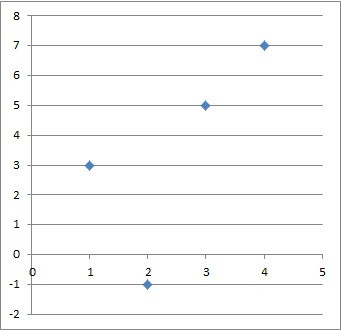The graph of the discrete function with four ordered pairs {(1, 3), (2, -1), (3, 5), (4, 7)}.

Other functions are continuous, since they are well-defined for an entire interval of real numbers.

For example, the function f(x) = x2 is continuous on the entire set of real numbers.  For any real number x, we just square the number to get the output, which will be another (nonnegative) real number.

You can see the graph of the continuous function f(x) = x2 below.

### Can Functions Be Both Even & Odd?

A function can be both even and odd in the special case of f(x) = 0.  Let’s use definitions and equations to prove why this is the only case.

Remember our definitions:

If a function f(x) is both even and odd, then both of the equations above are true.  This means that:

• f(-x) = f(-x)
• f(x) = f(-x)  [f(-x) = f(x) on the left side, since f(x) is even]
• f(x) = -f(x)  [f(-x) = -f(x) on the right side, since f(x) is odd]
• f(x) + f(x) = -f(x) + f(x)  [add f(x) to both sides]
• 2f(x) = 0
• f(x) = 0

So, f(x) = 0 is the only function that is both even and odd.

### Do Functions Have To Be One-To-One?

Functions do not have to be one-to-one.  Remember:

• A function passes the vertical line test, meaning that any vertical line x = a that you draw will intersect the graph of the function at most once.
• A one-to-one function passes the horizontal line test, meaning that any horizontal line y = b that you draw will intersect the graph of the function at most once.

A relation that passes the vertical line test is a function.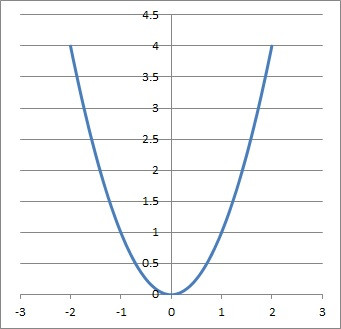The graph of f(x) = x2 is a function, since it passes the vertical line test. However, it is not one-to-one, since it fails the horizontal line test.

A function that passes the horizontal line test is a one-to-one function.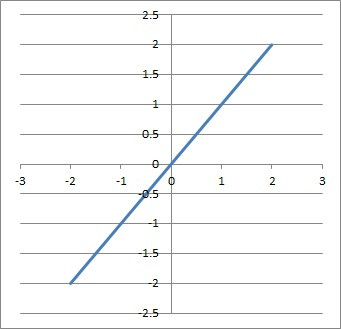The graph of (x) = x is a function, since it passes the vertical line test. It is a one-to-one function because it also passes the horizontal line test.

A function that is not one-to-one can have two inputs that lead to the same output. For example, f(x) = x2 is not one-to-one, since f(-2) = f(2) = 4 (both inputs x = -2 and x = 2 lead to an output of 4).

### When Is A Function Increasing (Or Decreasing)?

In algebraic terms:

• A function f(x) is increasing on an interval (a, b) if f(c) > f(d) when c > d for any c, d in (a, b).
• A function f(x) is decreasing on an interval (a, b) if f(c) < f(d) when c > d for any c, d in (a, b).

In calculus terms:

• A function f(x) is increasing on an interval (a, b) if f’(c) > 0 for any c in (a, b).
• A function f(x) is decreasing on an interval (a, b) if f’(c) < 0 for any c in (a, b).

In visual terms:

• A function f(x) is increasing on an interval (a, b) if the graph moves up at it moves right.
• A function f(x) is decreasing on an interval (a, b) if the graph moves down at it moves right.

Here are some examples of increasing and decreasing functions:

### When Is A Function Concave Up (Or Concave Down)?

We can use the 2nd derivative or the graph to tell us when a function is concave up or concave down.

In calculus terms:

• A function f(x) is concave up (convex) on an interval (a, b) if f’’(c) > 0 for any c in (a, b).
• A function f(x) is concave down (concave) on an interval (a, b) if f’’(c) < 0 for any c in (a, b).

In visual terms:

• A function f(x) is concave up (convex) on an interval (a, b) if the graph looks like a valley bottom.
• A function f(x) is concave down (concave) on an interval (a, b) if the graph looks like a mountain top.

Here are some examples of concave up and concave down functions:The function f(x) = x2 is concave up, since the graph looks like a valley bottom.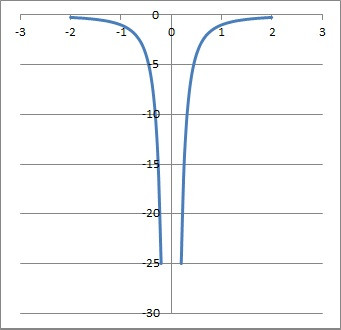The function f(x) = -1/x2 is concave down, since the graph looks like two mountain tops.

### Can Functions Cross Horizontal Asymptotes?

A function can cross a horizontal asymptote in some cases.  For example, take the function

• f(x) = (2x2 + 2x + 4)/(x2 – 1)

It has a horizontal asymptote at y = 2.  We can solve for y = 2 to find out the x-value where the function cross this asymptote:

• Y = 2
• f(x) = 2
• (2x2 + 2x + 4)/(x2 – 1) = 2
• 2x2 + 2x + 4 = 2(x2 – 1)
• 2x2 + 2x + 4 = 2x2 – 2
• 2x = -6
• x = -3

So, at x = -3, the value of f(x) is 2, meaning it crosses its own horizontal asymptote at (-3, 2).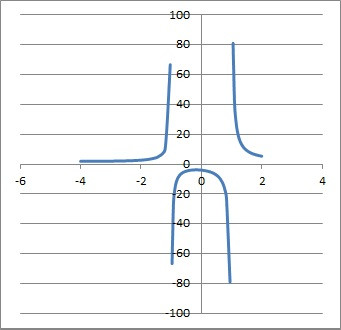The graph of the function (2x2 + 2x + 4)/(x2 – 1) crosses the horizontal asymptote y = 2 at the point (-3, 2).

### Can Functions Have Holes?

A function can have holes. This happens when the numerator and denominator of a rational function have the same linear factor.

For example, consider the function:

• f(x) = (x2 – 1) / (x – 1)

We can factor the numerator as a difference of squares, giving us:

• f(x) = (x + 1)(x – 1) / (x – 1)

So we have a factor of x – 1 in both the numerator and denominator. This means we will get a hole at x = 1 (which is where the factor x – 1 is zero).

Otherwise, the function behaves like y = x + 1 (which is what remains after we cancel the factors of x – 1).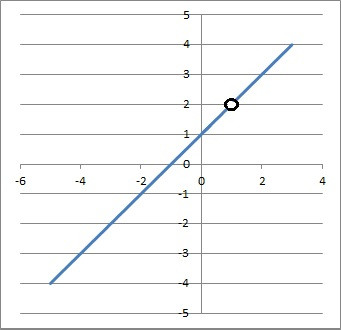The graph of the function f(x) = (x2 – 1) / (x – 1) has a hole at x = 1.

### Is A Function Continuous If It Is Differentiable?

A function is continuous if it is differentiable.  By definition, a function must be continuous at a point in order to be differentiable.

However, the opposite is not true: a continuous function may or may not be differentiable.